Algebra 2 2-4 Complete Lesson: More About Linear Equations
starstarstarstarstarstarstarstarstarstar
5 (1 rating)
by Matthew Richardson
| 36 Questions
Note from the author:
A complete formative lesson with embedded slideshow, mini lecture screencasts, checks for understanding, practice items, mixed review, and reflection. I create these assignments to supplement each lesson of Pearson's Common Core Edition Algebra 1, Algebra 2, and Geometry courses. See also mathquest.net and twitter.com/mathquestEDU.The outlined content above was added from outside of Formative.1
1
10 pts
Solve It! A contractor needs to build two straight roads, each passing through point A. One road must be parallel to Pine Street, and the other road must be perpendicular to Pine Street. Find the coordinates of a second point the parallel road will pass through and the coordinates of a third point the perpendicular road will pass through. Classify the coordinates below appropriately.
• (10, 70)
• (50, 40)
• (60, 60)
• On the parallel road
• On the perpendicular road
• On neither the parallel nor perpendicular road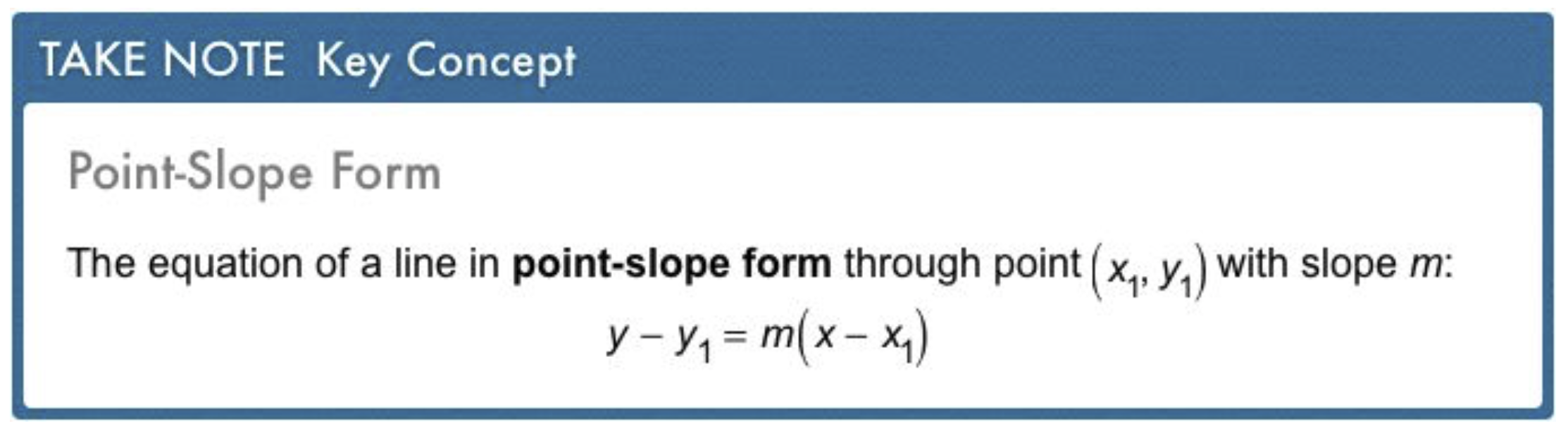2
2
10 pts
Problem 1 Got It?
A
B
C
D
3
10 pts
Problem 2 Got It? A line passes through (-5, 0) and (0, 7). What is an equation of the line in point-slope form?

4
10 pts
Problem 2 Got It? Reasoning: What is another equation in point-slope form of the line through the points (-5, 0) and (0, 7)?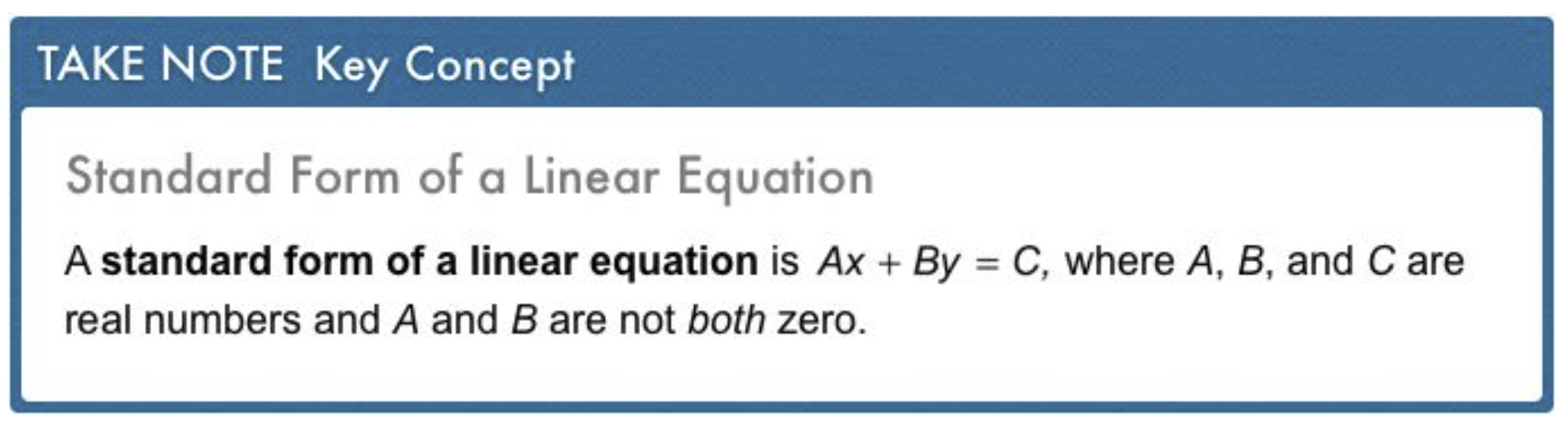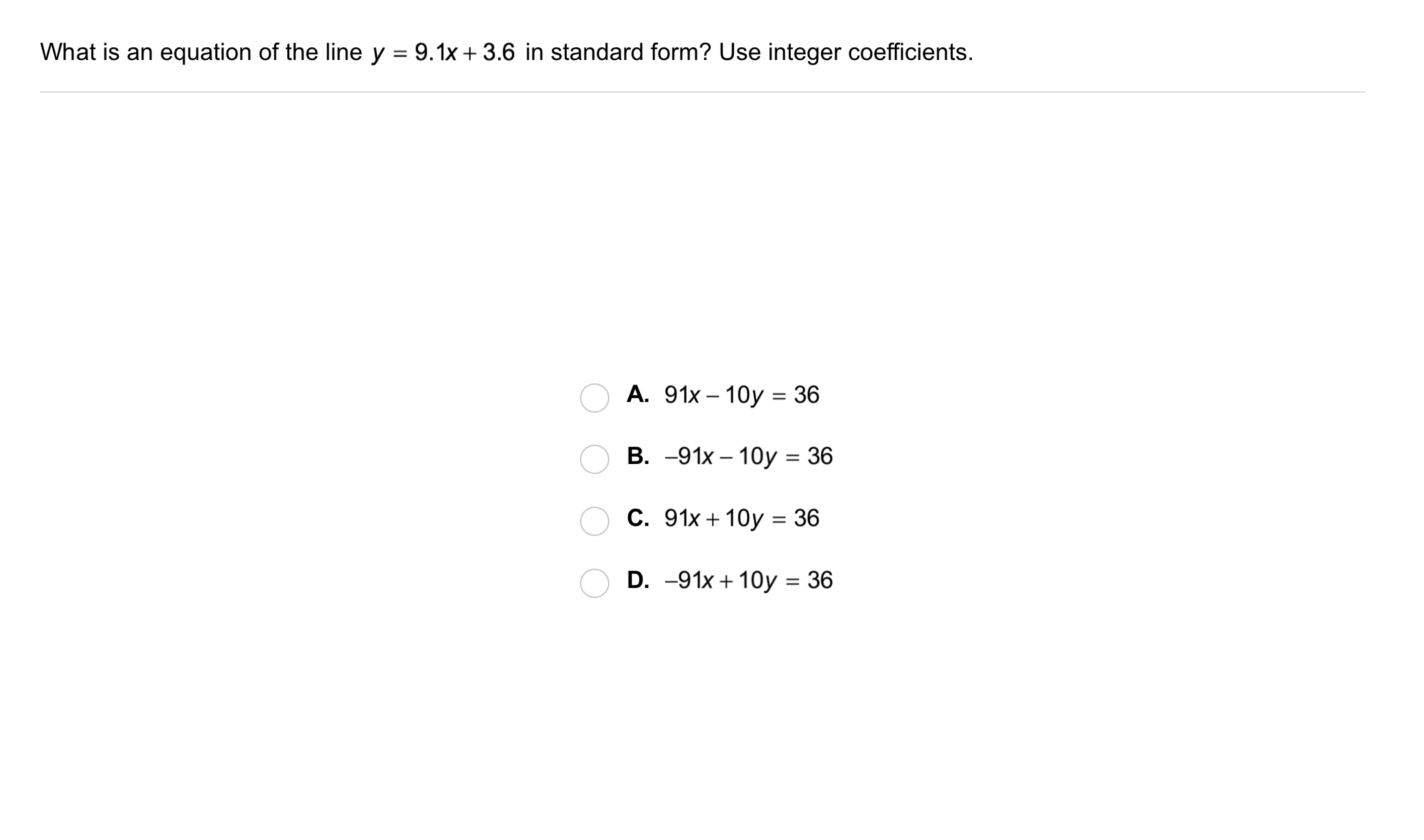5
5
10 pts
Problem 3 Got It?
A
B
C
D6
10 pts
Problem 4 Got It? What are the intercepts of 2x - 4y = 8?
• (0, 0)
• (4, 0)
• (0, -2)
• (-4, 0)
• x-intercept
• y-intercept
7
10 pts
Problem 4 Got It? Graph the equation 2x - 4y = 8 on the canvas. Use a color other than black.
8
10 pts
Problem 4 Got It? Graph the equation. Zoom and pan your graph to leave an appropriate scale and viewing window.
You may edit your own graph on the previous item after completing this item.
9
10 pts
Problem 5 Got It? The office manager of a small office ordered 140 packs of printer paper. Based on average daily use, she know that the paper will last about 80 days.

What graph represents this situation? Zoom and pan your graph to leave an appropriate scale and viewing window.
10
10 pts
Problem 5 Got It? The office manager of a small office ordered 140 packs of printer paper. Based on average daily use, she know that the paper will last about 80 days.

What is the equation of the line in standard form?
4y - 7x = 560
7x + 4y = 560
7x + 80y = 560
11
10 pts
Problem 5 Got It? The office manager of a small office ordered 140 packs of printer paper. Based on average daily use, she know that the paper will last about 80 days.

How many packs of printer paper should the manager expect to have after 30 days?
87.5 packs
31.5 packs
37 packs
19 packs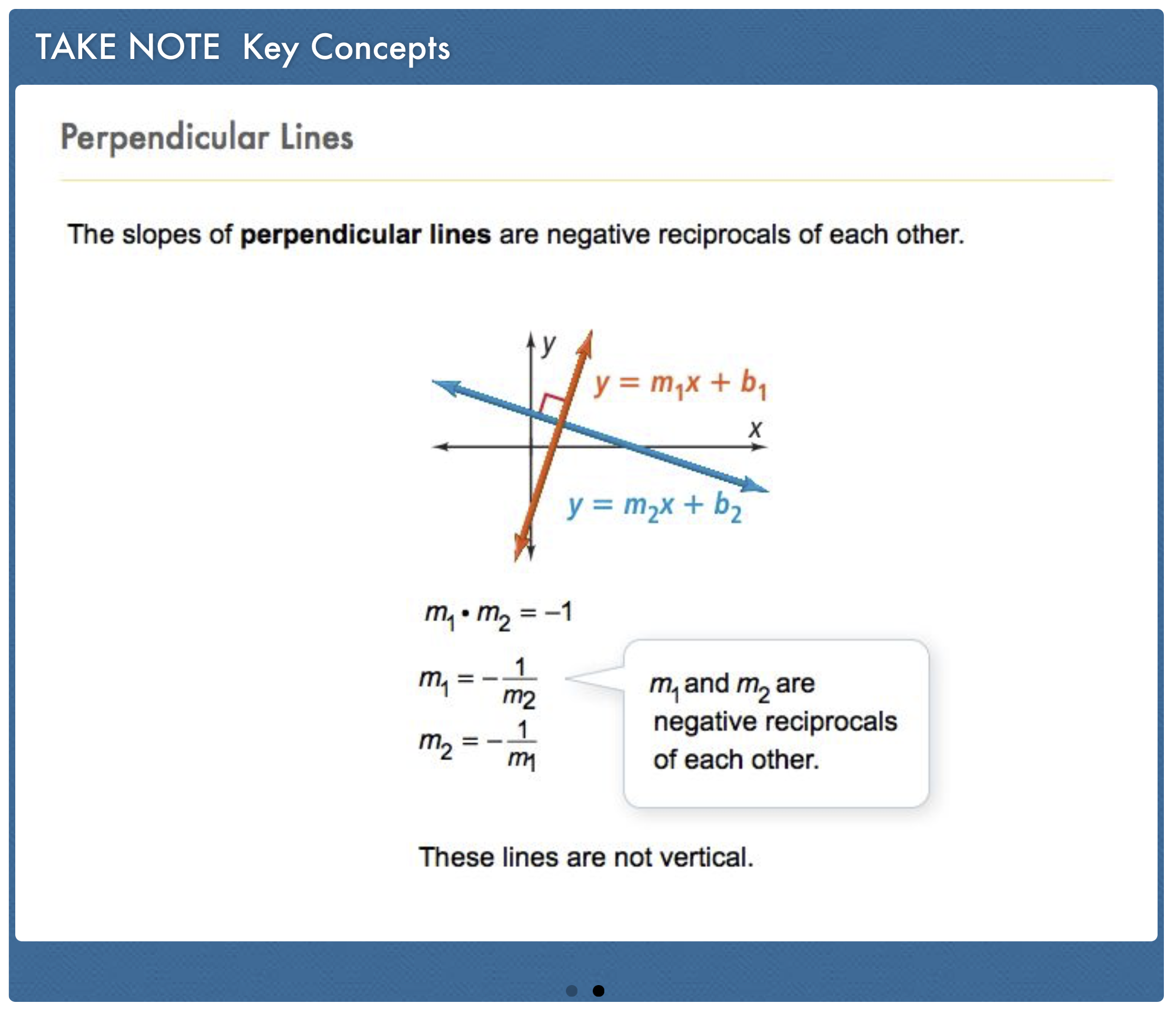12
12
10 pts
Problem 6 Got It?
A
B
C
D13
13
10 pts
Problem 6 Got It?
A
B
C
D14
14
10 pts
A
B
C
D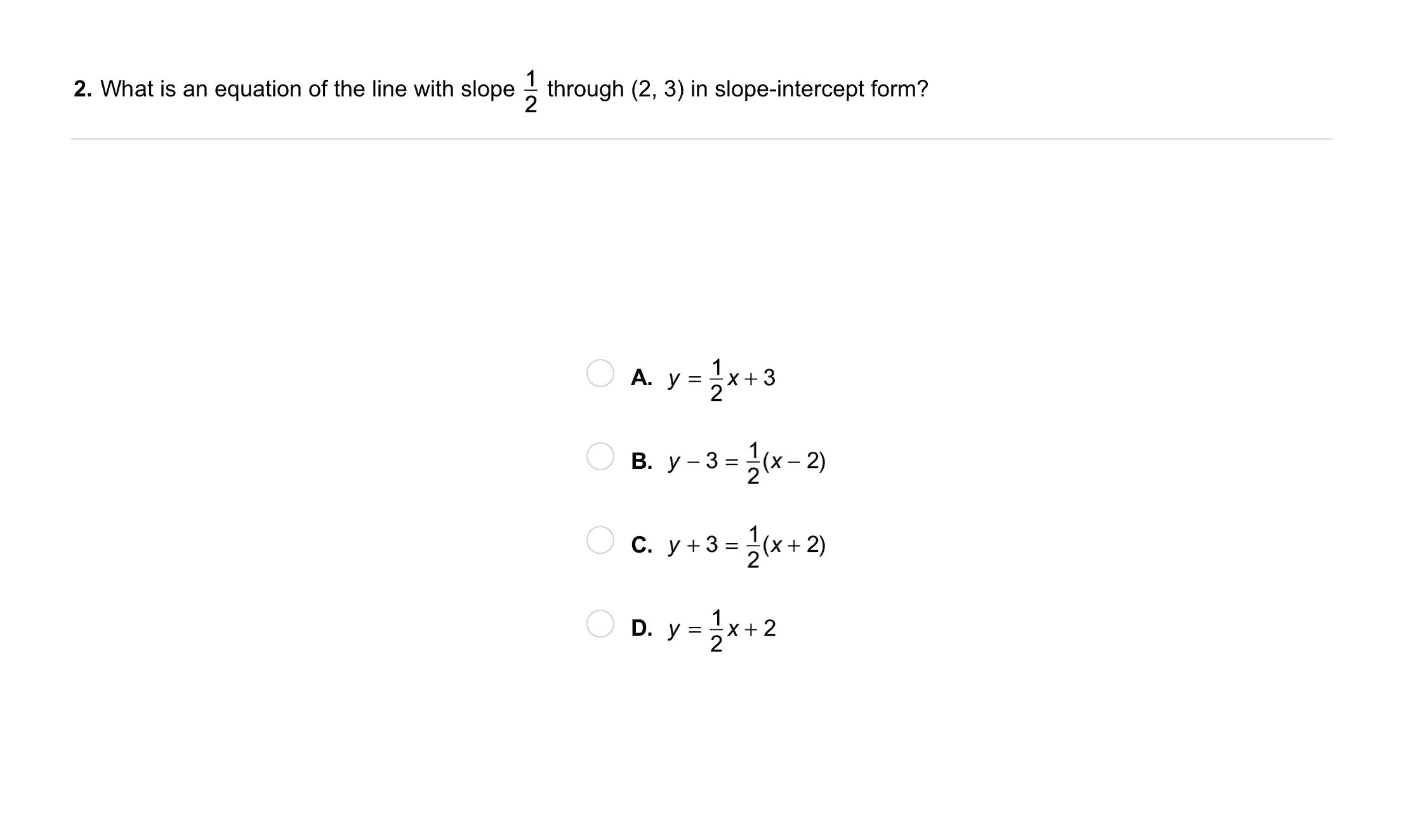15
15
10 pts
A
B
C
D
16
30 pts
Graphing: What are the intercepts of 3x + y = 6? Graph the equation. Be sure to include relevant graph detail: label axes, indicate units and scale on both axes, and use arrows to represent end behavior, as appropriate.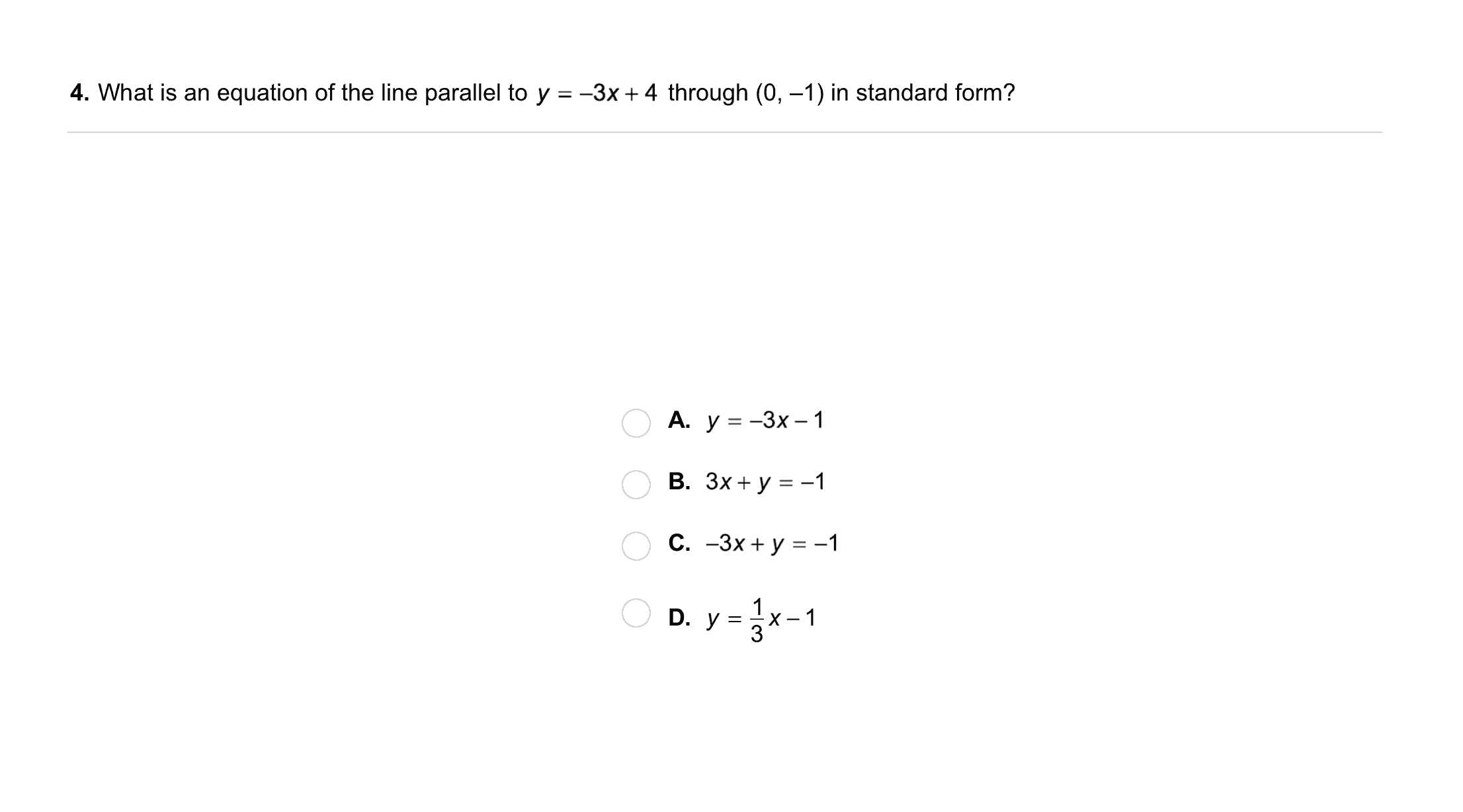17
17
10 pts
A
B
C
D18
18
10 pts
A
B
C
D19
10 pts
Vocabulary: Tell whether the equation is in slope-intercept, point-slope, or standard form.
y + 2 = -2(x - 1)
standard form
slope-intercept form
point-slope form
20
10 pts
Vocabulary: Tell whether the equation is in slope-intercept, point-slope, or standard form.
y = -¼x + 9
slope-intercept form
standard form
point-slope form
21
10 pts
Vocabulary: Tell whether the equation is in slope-intercept, point-slope, or standard form.
-x - 2y = 1
point-slope form
slope-intercept form
standard form
22
10 pts
Vocabulary: Tell whether the equation is in slope-intercept, point-slope, or standard form.
y - 3 = 4x
standard form
point-slope form
slope-intercept form
23
10 pts
Understanding: Which form would you use to write the equation of a line if you knew its slope and x-intercept? Explain.
24
10 pts
Understanding: If the intercepts of a line are (a, 0) and (0, b), what is the slope of the line? Assume that a and b are both greater than 0.

Enter only the slope, in simplified fraction form.
25
10 pts
Error Analysis: Your friend says the line y = -2x + 3 is perpendicular to the line x + 2y = 8. Do you agree? Explain.
No; After converting the second equation to slope-intercept form, we can see that its slope is -1/2. Since -2 and -1/2 are not opposite reciprocals, the lines are not perpendicular.
Yes; After converting the second equation to slope-intercept form, we can see that its slope is 1/2. Since -2 and 1/2 are opposite reciprocals, the lines are perpendicular.26
10 pts
Review Lesson 2-1: Match each relation with its domain.
• {(-3, 4), (-1, 2), (0, -2), (1, 0), (2, 2)}

• Domain: all real numbers
• Domain: {-3, -1, 0, 1, 2}
• Domain: {-18, -2, 0, 3, 39}
27
10 pts
Review Lesson 2-1: Match each relation with its range.
• {(-3, 4), (-1, 2), (0, -2), (1, 0), (2, 2)}

• Range: {-2}
• Range: {-1, 3, 17, 28, 32}
• Range: {-2, 0, 2, 4}
28
10 pts
Review Lesson 2-1: Determine whether each relation represents a function.
• {(-3, 4), (-1, 2), (0, -2), (1, 0), (2, 2)}

• Function
• Not a function
29
10 pts
Review Lesson 1-2: Match each equation with the property of real numbers it illustrates.

• Distributive property
• Inverse property of multiplication
• Inverse property of addition
30
10 pts
Review Lesson 2-3: Match the equation of each line in slope-intercept form with its corresponding details on the right.

• m = 3 and the y-intercept is (0, -5)
• m = 1/2 and the y-intercept is (0, 0)
• m = -3/8 and the y-intercept is (0, 3)31
10 pts
Vocabulary Review: Write the sentence below as an equation.

The sum of x and y is 8.
32
10 pts
Vocabulary Review: Write the sentence below as an equation.

The product of p and q is 7.
33
10 pts
Vocabulary Review: Write the sentence below as an equation.

The square root of y is x cubed.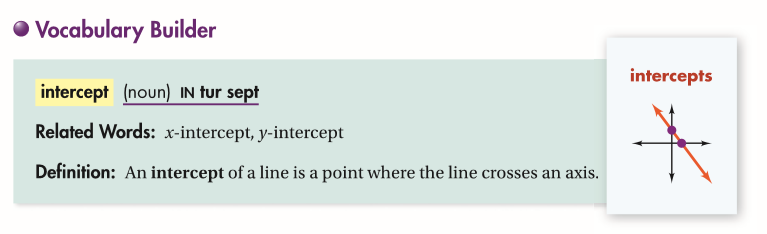34
10 pts
Use Your Vocabulary: Use the graph below to categorize each statement as True or False.
• The x-intercept of the line is (4, 0).
• The x-intercept of the line is (0, 4).
• The y-intercept of the line is (-1, 0).
• The y-intercept of the line is (0, -1).
• True
• False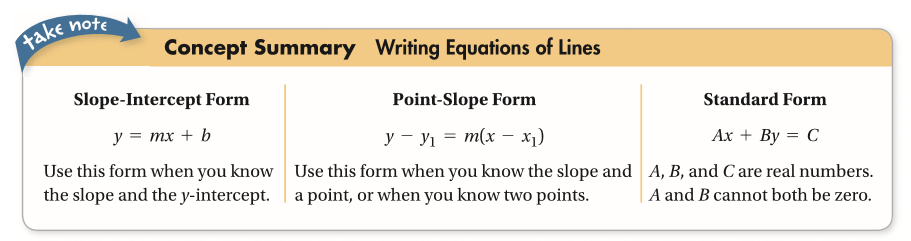35
100 pts
Notes: Take a clear picture or screenshot of your Cornell notes for this lesson. Upload it to the canvas. Zoom and pan as needed.

For a refresher on the Cornell note-taking system, click here.
36
10 pts
Reflection: Math Success
Add to my formatives list

Formative uses cookies to allow us to better understand how the site is used. By continuing to use this site, you consent to the Terms of Service and Privacy Policy.